# Consistent Units in Abaqus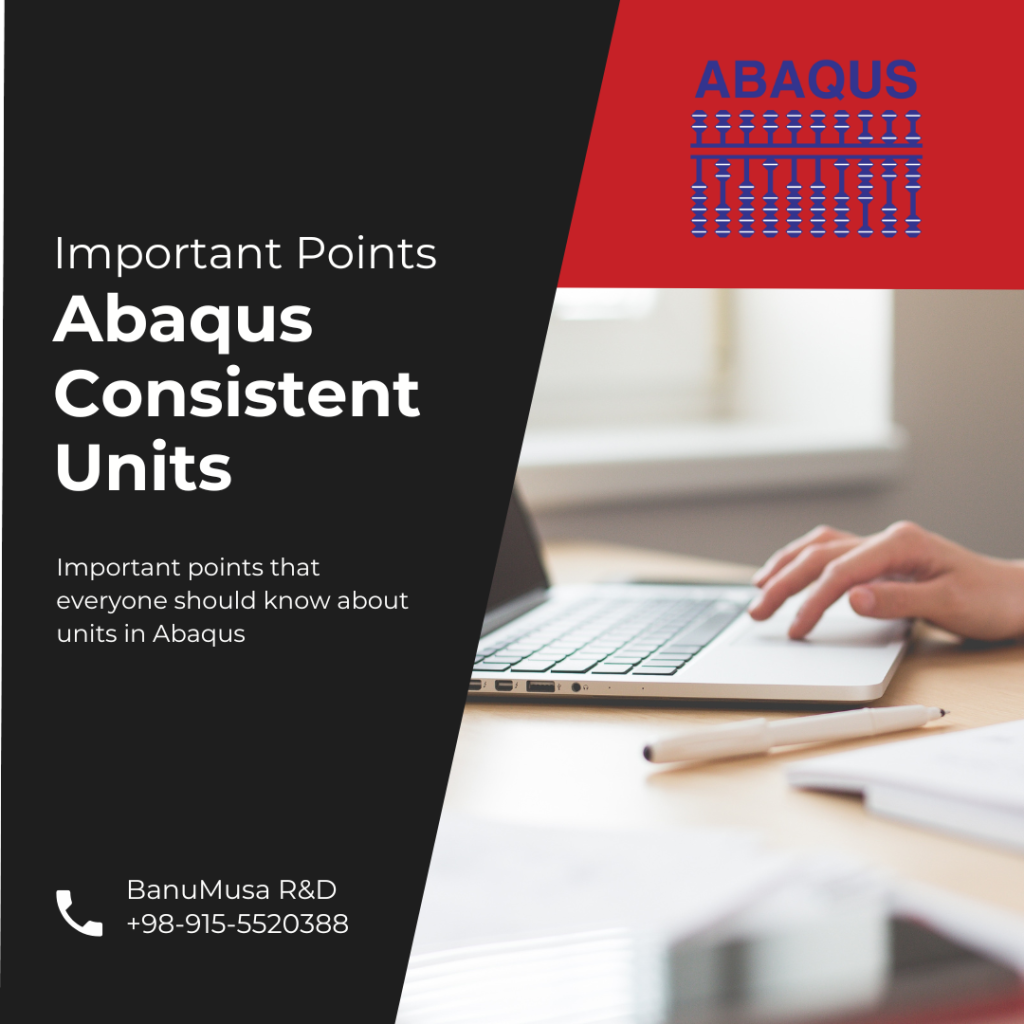# Abaqus Consistent Units

For beginners or even experienced users of Abaqus, the question arises of “how to convert units in Abaqus?”, “What unit system is used in Abaqus?”, “How to check the unit system in Abaqus? And questions like this. In this post, we will explain the unit system in Abaqus from A to Z! It is essential which unit system is used in an impact or static analysis. The SI unit system, or Imperial system, offers a set of units for mass, length, and time that other quantities can use with known and conventional units. Although this may seem simple, the conclusions that will be drawn from it are very important and interesting results that will help us to better model and analysis. In the SI system, for example, force, pressure, and other quantities can be easily measured.

F=m.a è Kg . m/s^2 =N

## Value of units

With a little care, we can say that the units that have more value are larger. For example, the value of the MPa unit for pressure is 10.6 times the Pa unit. The question may arise that if in analysis the units are based on mm, will the unit of pressure be MPa? If the unit of force is Newton, the answer is yes.

Is it possible to select the unit of mass and time in such a way that the force is obtained based on the same force? The answer is yes. I have explained below.

# Consistent units

If the unit of time is seconds and the unit of length is millimeters, what unit must be chosen for the mass so that the force is the same as Newton’s?

To do this, you must do the following. If X is a new unit of mass and its relative value is compared to kilograms (X = a Kg):

N = (a Kg) mm/s^2 = (a Kg) 10e-3 m/s^2 = (10e-3 (a) (Kg.m/s^2) = (10e-3 a)(N)

The quantity (10 e-3 a) must be equal to one to obtain the force based on Newton. Therefore, the new unit of mass in this case should be 1000 kg or one ton.

Because Abaqus usually works with the density, for a material such as steel, the density must be entered in tons per cubic millimeter.

7800 Kg/m^3 = 7800 . 10^-3 ton/10^9 mm^3 = (7800). 10^-12 ton/mm^3

Poor selection of consistent units sometimes causes problems for the analyst. Consider the following example.

If mass is in kilograms and length is in millimeters, the unit of time b sec can be obtained in such a way that the force is obtained in Newtons:Therefore, the coefficient must be  equal to one.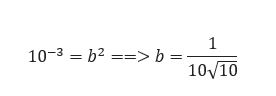That is, in this consistent but unsuitable system, the unit of time will be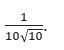In special cases, such as geological problems where very large masses travel very short distances over very long periods of time – for example, the movement of the Earth’s crust over 100 million years – it is best to use the following consistent units.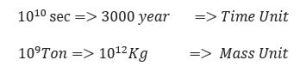If the meter unit is selected for length, the necessary operations can be easily performed to identify the force unit.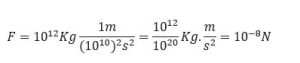This change of units makes analysis and modeling easier.

## Other widely used units system

In addition to the SI m/mm system, cgs is another widely used unit system. In this system, length is in centimeters, mass in grams, and time in seconds.

The cm.g.micro s unit system is another unit system commonly used for impact and explosion analysis. In case of impact due to the application of high forces in a short time, this system will be very suitable for compatible units. If other units such as kilograms, meters, and seconds are used, there is a possibility of error due to rounding numbers with a high number of decimals (per unit time).

The table below shows the different modes of compatible units that you can always use to check units and prevent modeling errors.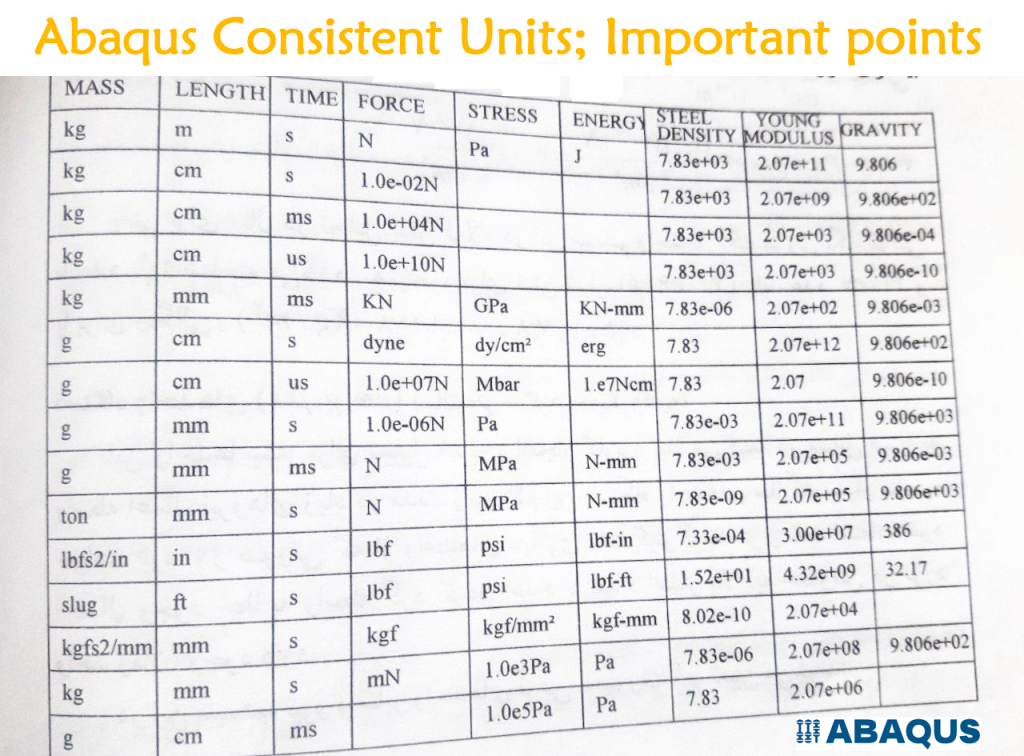## Online Abaqus Units ConversionBut Carl Osterwisch has made things a lot easier. You can convert different dimensions to different unit systems much easier and faster online. Just click on this link

## Material data Units Conversion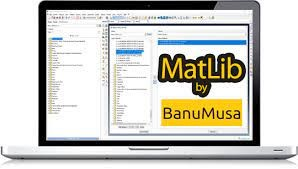At BanuMusa R&D, we have also prepared a comprehensive database of all high-consumption materials in simulation for the convenience of Abaqus users. You can read more information here

One of the features of this plugin is no need to convert material specification units. If you work with SI m/mm or English system. All material data has already been converted.

## Convert Units Plugin

And finally, there is a plugin for converting units in Abaqus that you can download through this link which is developed by James Lockley in 2005. It’s not a perfect plugin, but it’s a good start.

I hope you will use Abaqus better from now on…

5 1 vote
Article Rating
Subscribe
Notify of1 Comment
Inline Feedbacks